# Precalculus - Ellipses

## Introduction

• Ellipse is the set of all points in a plane such the sum of the distances from two fixed points, called the foci, is constant.
• An ellipse is formed when the cone is cut obliquely to the axis and the surface of the cone.
• To roughly visualize an ellipse, you can imagine two parabolas joined together.
• Study the graph of the ellipse below.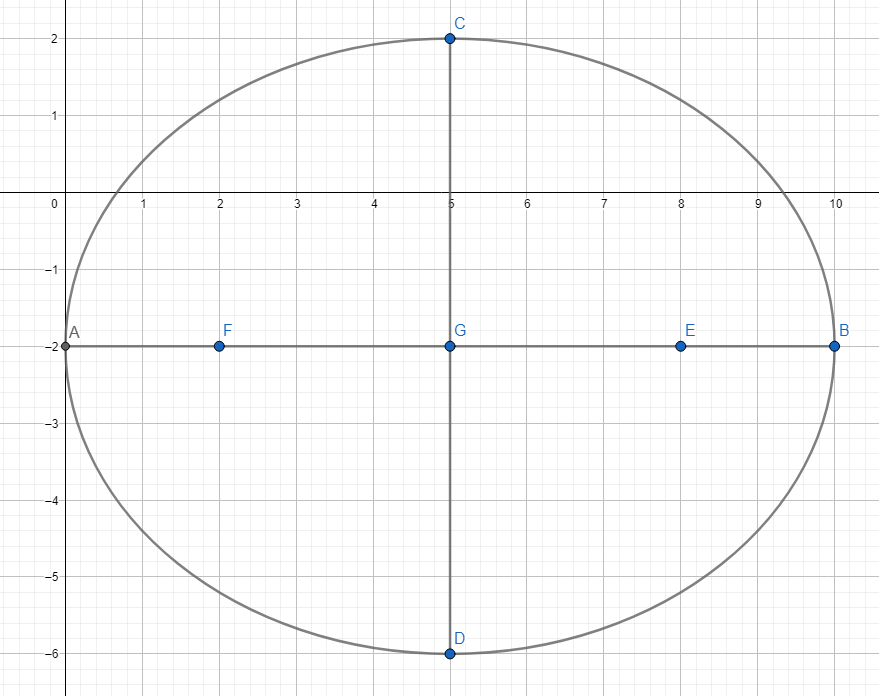• The graph of an ellipse crosses the major axis at two fixed points called vertices typically written in symbols . The ellipse above has vertices at .
• The coordinates of the center are at $C\left(h,k\right)=C\left(5,-2\right)$.
• The major axis in the example above is the line segment $AB$ while the minor axis is the line segment $CD$. The minor axis is the line segment through the center perpendicular to the major axis.
• The length of the major axis is given by ${d}_{1}+{d}_{2}=2a$. In the case above, .
• The coordinates of the foci are typically given by the symbols if the major axis is parallel to the x-axis. In the example above, the coordinates of the foci are .
• The line segment through the coordinates of the foci is called the latus rectum (focal chord). The length of the latus rectum is given by $L=\frac{2{b}^{2}}{a}$.
• The eccentricity of the ellipse is $0 or $e=\frac{c}{a}$.
• The general equation of the ellipse is given by .
• The discriminant of an ellipse is .
• The circumference of an ellipse is given by $C=2\mathrm{\pi }\left(\sqrt{\frac{{\mathrm{a}}^{2}+{\mathrm{b}}^{2}}{2}}\right)$ and the area of the ellipse is given by $A=\mathrm{\pi }\sqrt{\mathrm{AC}}$.

## Forms and Graphs of Ellipse

1. Center at $C\left(0,0\right)$ with major axis = x-axis and minor axis = y-axis

Graph: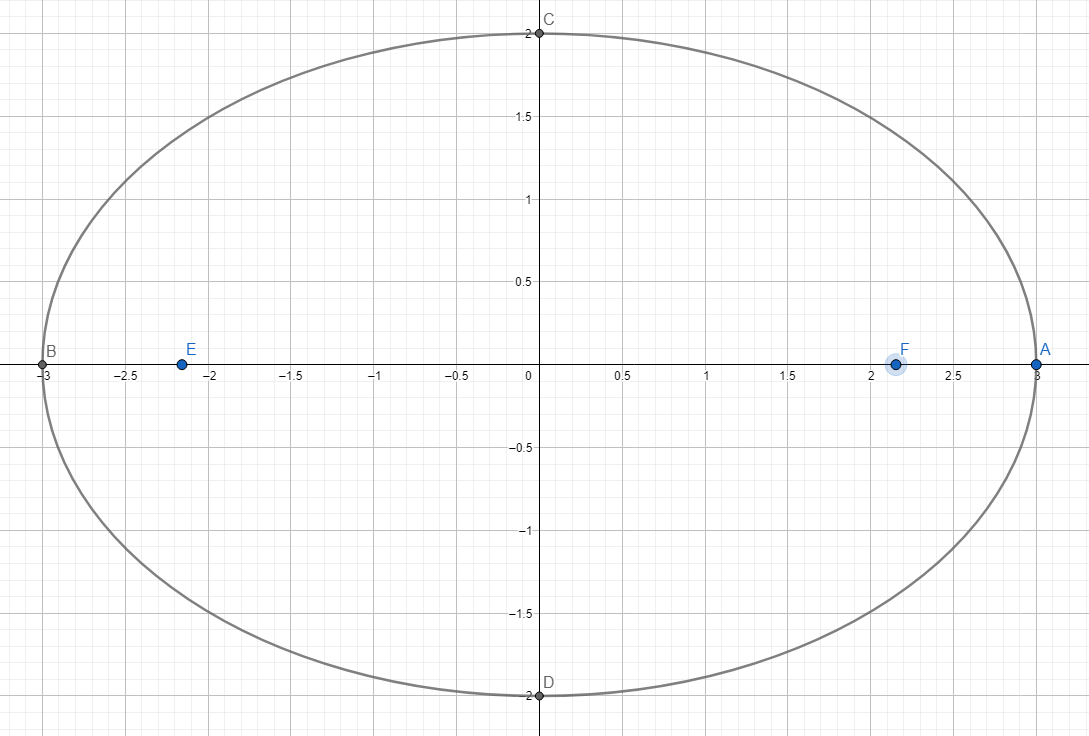Standard Equation is

To find the value of a, b, or c, we use ${a}^{2}={b}^{2}+{c}^{2}$.

The coordinates of the vertices are .

Co-vertices are .

The coordinates of the foci are .

The equation of the directrix is given by $x=±\frac{a}{e}$.

2. Center at $C\left(0,0\right)$ with major axis = y-axis and minor axis = x-axis.

Graph: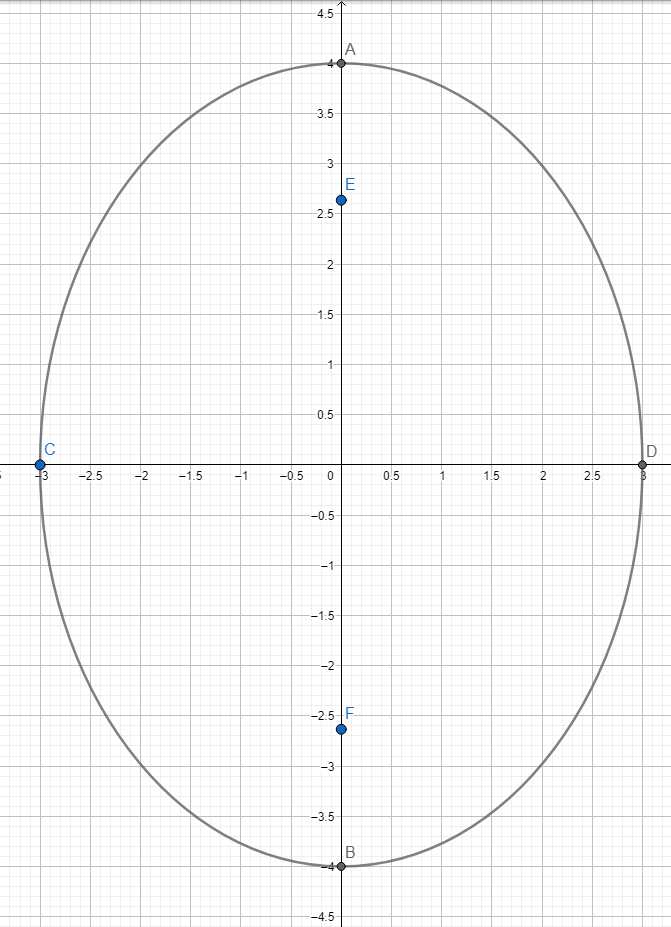Standard Equation is $\frac{{x}^{2}}{{b}^{2}}+\frac{{y}^{2}}{{a}^{2}}=1$ where $a>b$.

To find the value of a, b, or c, we use ${a}^{2}={b}^{2}+{c}^{2}$.

The coordinates of the vertices are .

Co-vertices are .

The coordinates of the foci are .

The equation of the directrix is given by $y=±\frac{a}{e}$.

3. Center at $C\left(h,k\right)$, major axis is parallel to the x-axis, and the minor axis is parallel to the y-axis.

Graph: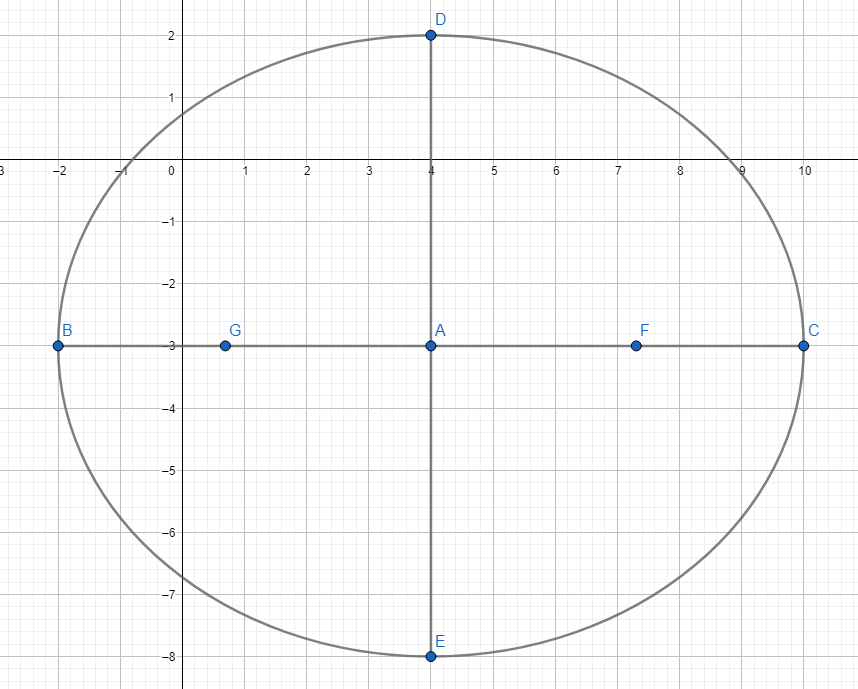The standard Equation is $\frac{{\left(x-h\right)}^{2}}{{a}^{2}}+\frac{{\left(y-k\right)}^{2}}{{b}^{2}}=1$ where $a>b$

To find the value of a, b, or c, we use ${a}^{2}={b}^{2}+{c}^{2}$

The coordinates of the vertices are .

Co-vertices are

The coordinates of the foci are

The equation of the directrix is $x=h±\frac{{a}^{2}}{c}$

4. Center at $C\left(h,k\right)$, the major axis is parallel to the y-axis, and the minor axis is parallel to the x-axis.

Graph: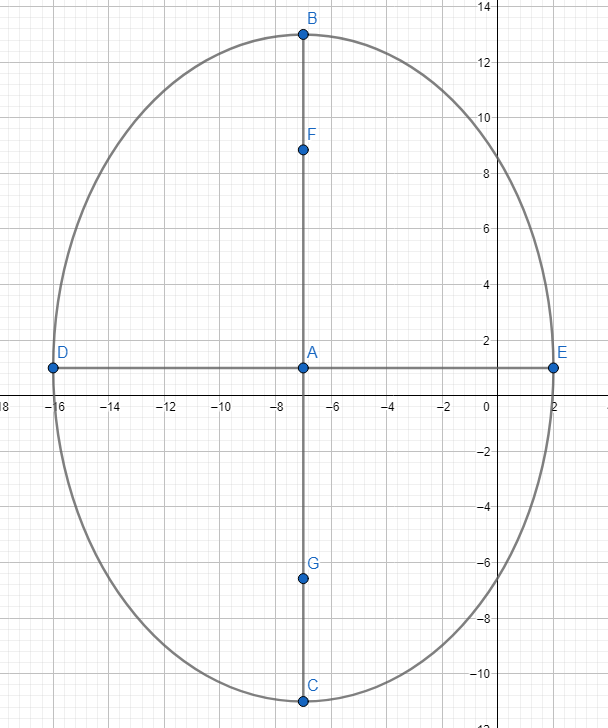Standard equation is

To find the value of a, b, or c, we use ${a}^{2}={b}^{2}+{c}^{2}$.

The coordinates of the vertices are .

Co-vertices are

The coordinates of the foci are

The equation of the directrix is $y=k±\frac{{a}^{2}}{c}$

## Solved Examples

Example 1. Given the ellipse $\frac{{\left(x+9\right)}^{2}}{121}+\frac{{\left(y-3\right)}^{2}}{81}=1$, find the coordinates of the center, foci, and vertices.

Solution:

The major axis is parallel to the x-axis.

Based on the given equation, we have . Thus, the center is at $C\left(-9,3\right)$.

To solve for $a$, we look for the larger denominator and take its square root. Hence, $a=11$ and $b=9$.

To solve for $c$, we use ${a}^{2}={b}^{2}+{c}^{2}$.

${\left(11\right)}^{2}={\left(9\right)}^{2}+{c}^{2}$

$c=2\sqrt{10}$

The coordinates of the foci are .

The coordinates of the vertices are

Example 2. Find the equation of the ellipse with foci  and a major axis length of 34 units.

Solution:

By inspection of the given points, the ellipse has a major axis parallel to the y-axis. It follows the standard equation .

To solve for a, we have:

$2a=34$

$a=17$

To solve for c, we use the y-coordinates of the foci.

$\left\{\begin{array}{l}k-c=-9\\ k+c=7\end{array}\right\$

Solving the system yields .

To solve for b, we use ${a}^{2}={b}^{2}+{c}^{2}$.

${\left(17\right)}^{2}={b}^{2}+{\left(8\right)}^{2}$

${b}^{2}=225\to b=15$

Hence, the equation of the ellipse is $\frac{{\left(x-4\right)}^{2}}{225}+\frac{{\left(y+1\right)}^{2}}{289}=1$.

Example 3. Write the equation of the ellipse ${x}^{2}+2{y}^{2}-6x+20y+41=0$ in standard form.

Solution:

Use completing the square. To illustrate, we have:

${x}^{2}-6x+2{y}^{2}+20y=-41$

${x}^{2}-6x+9+2\left({y}^{2}+10y+25\right)=-41+9+2\left(25\right)$

${\left(x-3\right)}^{2}+2{\left(y+5\right)}^{2}=18\to \frac{{\left(x-3\right)}^{2}}{18}+\frac{{\left(y+5\right)}^{2}}{9}$

## Cheat Sheet

• The ellipse $\frac{{\left(x-7\right)}^{2}}{12}+\frac{{\left(y-2\right)}^{2}}{8}=1$ has its center at $C\left(7,2\right)$ with a major axis parallel to the x-axis.
• The ellipse $\frac{{\left(x+\frac{3}{4}\right)}^{2}}{225}+\frac{{\left(y-2\right)}^{2}}{400}=1$ has its center at $C\left(-\frac{3}{4},2\right)$ with a major axis parallel to the y-axis.
• The ellipse in the form of $\frac{{\left(x-h\right)}^{2}}{{a}^{2}}+\frac{{\left(y-k\right)}^{2}}{{b}^{2}}=1$ has a horizontal major axis.
• The ellipse $\frac{{\left(x-h\right)}^{2}}{{b}^{2}}+\frac{{\left(y-k\right)}^{2}}{{a}^{2}}=1$ has a vertical major axis.
• Always be careful of the sign when dealing with coordinates of the center, foci, vertices, and co-vertices.
• Be mindful of the difference between .

## Blunder Areas

• Don't be confused with the equation ${a}^{2}={b}^{2}+{c}^{2}$ and the Pythagorean Theorem ${c}^{2}={a}^{2}+{b}^{2}$
• It is a common mistake to identify cases like $\frac{{\left(x+3\right)}^{2}}{18}+\frac{{\left(y+5\right)}^{2}}{20}=1$ has their center at $C\left(3,5\right)$. The correct coordinates of the center is $C\left(-3,-5\right)$.
• To avoid confusion in solving problems involving ellipses, always check or inspect the points given. The coordinates and location of the center, foci, vertices, and co-vertices determine the type of ellipse and its major axis.
• Be careful in using the values of $a$ and $b$ for the equation $\frac{{\left(x-h\right)}^{2}}{{a}^{2}}+\frac{{\left(y-k\right)}^{2}}{{b}^{2}}=1$. It is by default that $a>b.$ The position of  changes depending on the major axis.